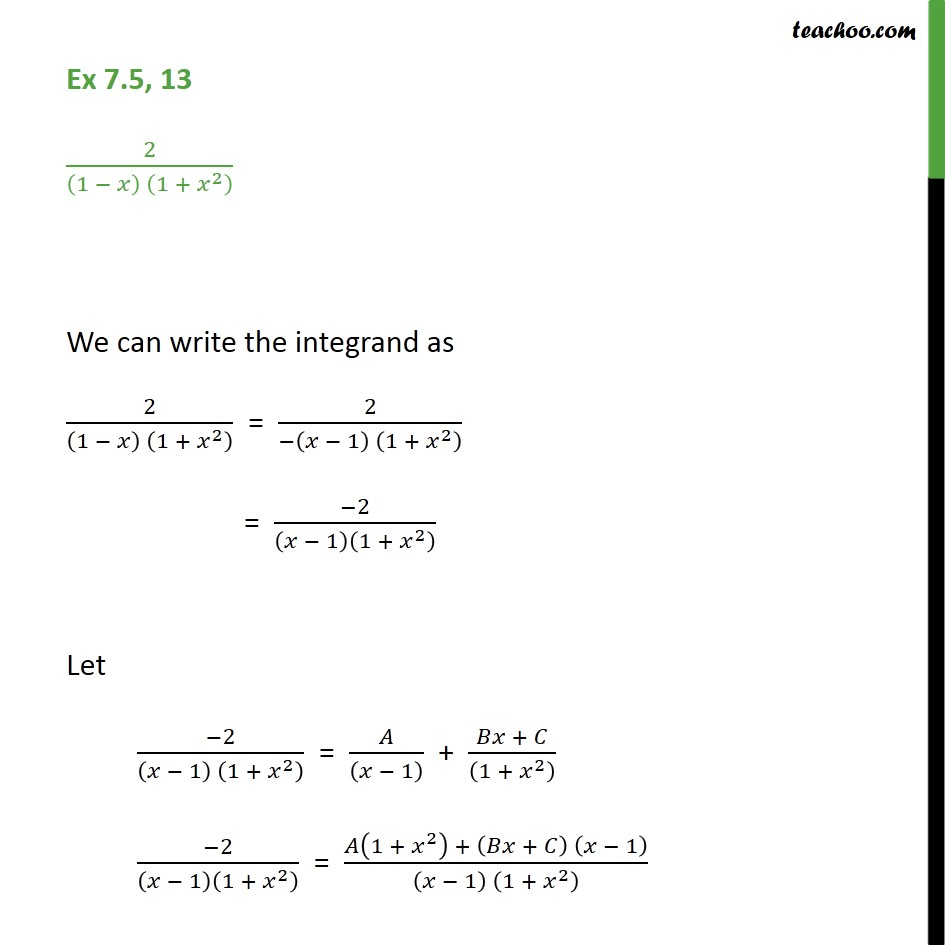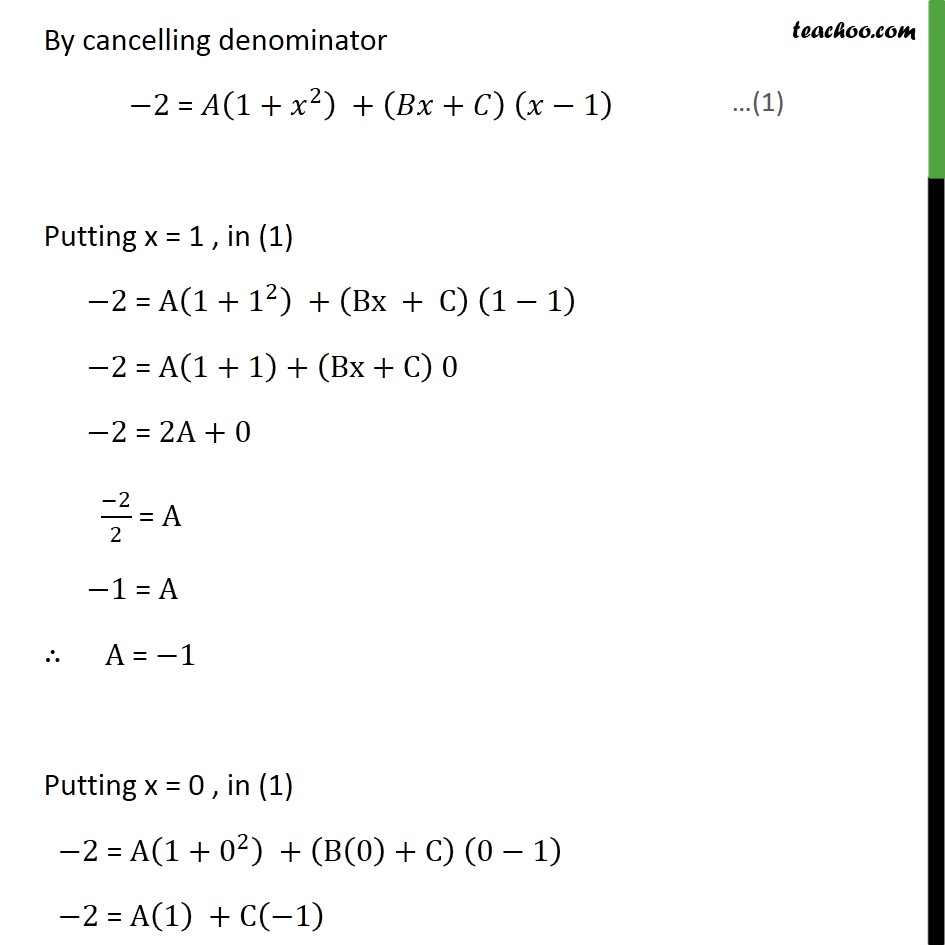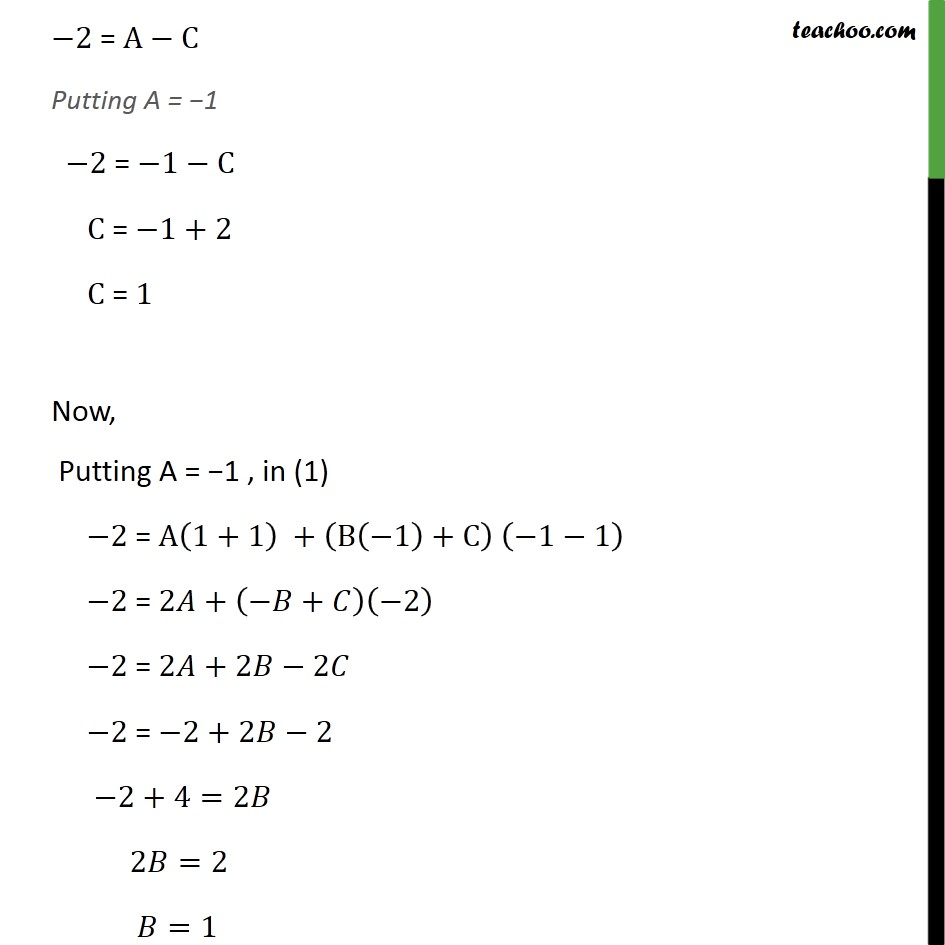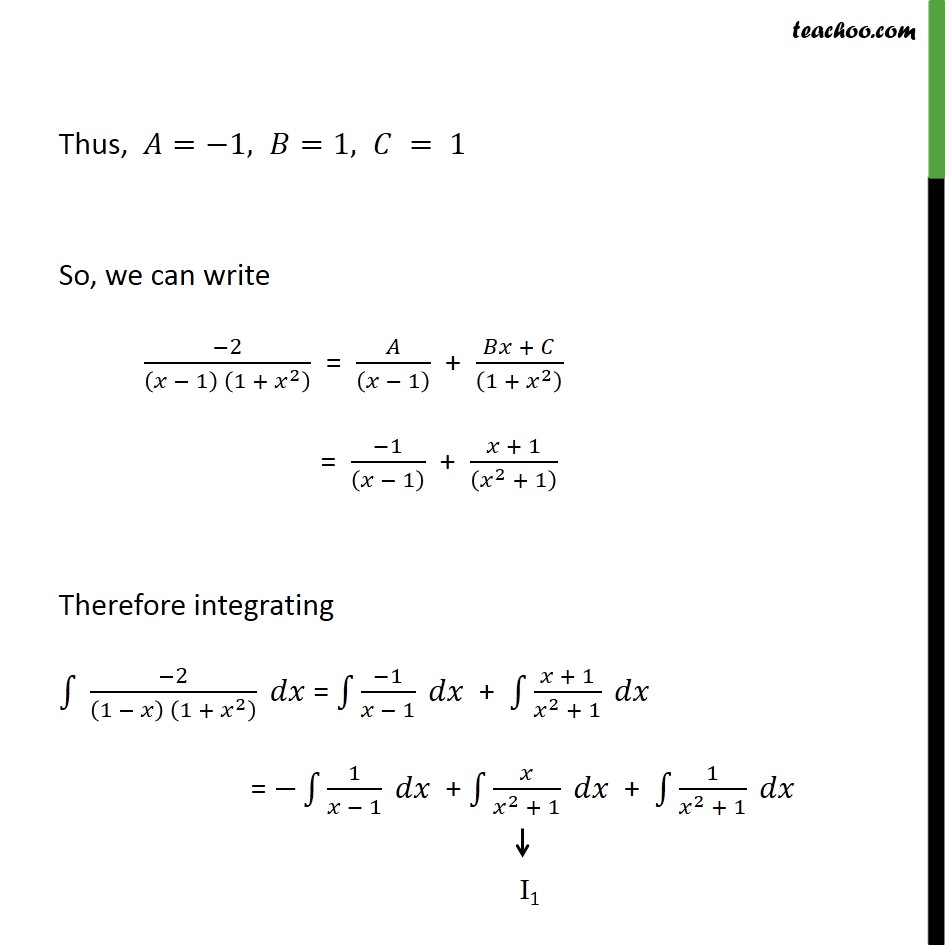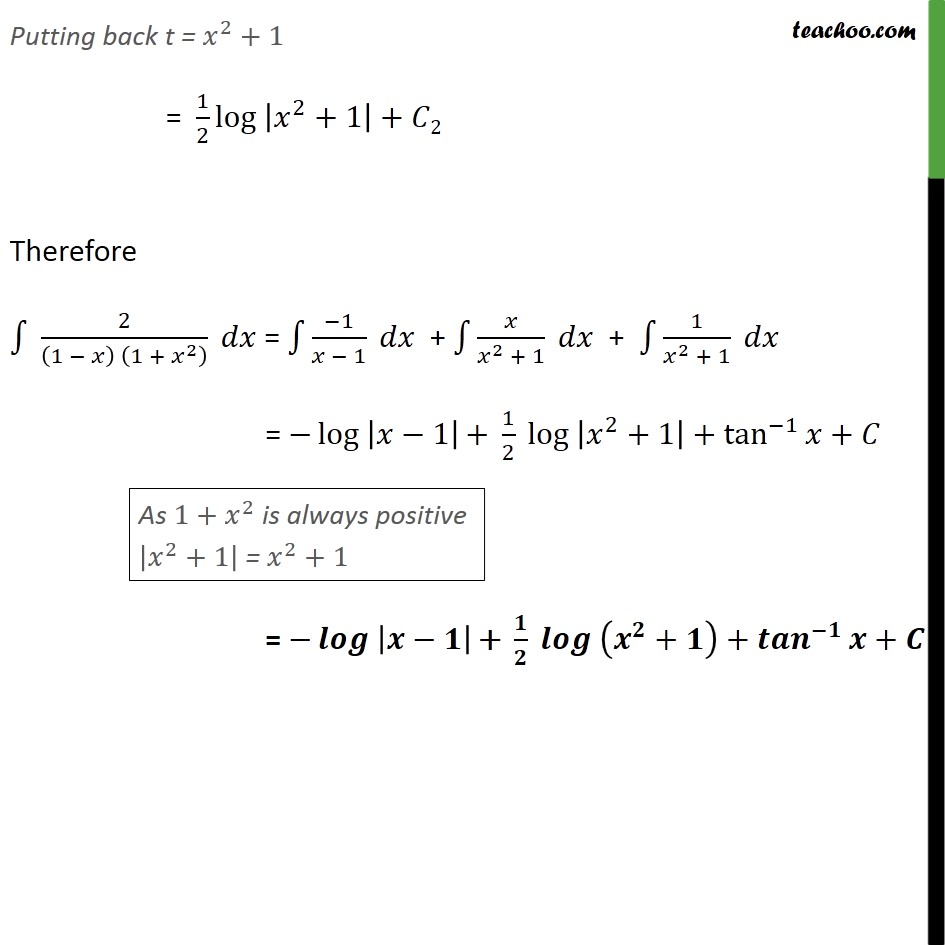1. Chapter 7 Class 12 Integrals
2. Concept wise
3. Integration by partial fraction - Type 5

Transcript

Ex 7.5, 13 2 1 1 + 2 We can write the integrand as 2 1 1 + 2 = 2 1 1 + 2 = 2 1 1 + 2 Let 2 1 1 + 2 = 1 + + 1 + 2 2 1 1 + 2 = 1 + 2 + + 1 1 1 + 2 By cancelling denominator 2 = 1+ 2 + + 1 Putting x = 1 , in (1) 2 = A 1+ 1 2 + Bx + C 1 1 2 = A 1+1 + Bx+C 0 2 = 2A+0 2 2 = A 1 = A A = 1 Putting x = 0 , in (1) 2 = A 1+ 0 2 + B 0 +C 0 1 2 = A 1 +C 1 2 = A C Putting A = 1 2 = 1 C C = 1+2 C = 1 Now, Putting A = 1 , in (1) 2 = A 1+1 + B 1 +C 1 1 2 = 2 + + 2 2 = 2 +2 2 2 = 2+2 2 2+4=2 2 =2 =1 Thus, = 1, =1, = 1 So, we can write 2 1 1 + 2 = 1 + + 1 + 2 = 1 1 + + 1 2 + 1 Therefore integrating 2 1 1 + 2 = 1 1 + + 1 2 + 1 = 1 1 + 2 + 1 + 1 2 + 1 Solving 1 I2 = 2 + 1 Let = 2 +1 = 2 2 = Hence 2 + 1 = . 2 = 2 = 1 2 log + 1 Putting back t = 2 +1 = 1 2 log 2 +1 + 2 Therefore 2 1 1 + 2 = 1 1 + 2 + 1 + 1 2 + 1 = log 1 + 1 2 log 2 +1 + tan 1 + = + + + +

Integration by partial fraction - Type 5